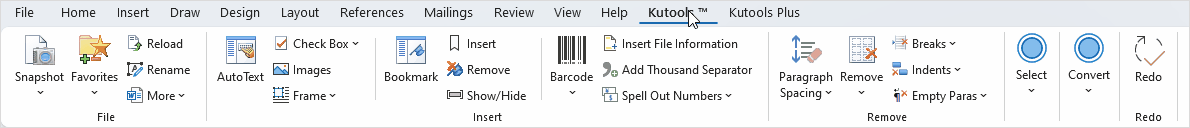## Quickly convert text to equation captions in Word

### Kutools for Word

Kutools for Word's Rebuild Equation Caption utility is able to convert text to equation captions quickly if the text is formatted as caption but it’s not a caption yet and it locates above or below the equations. If the original equation caption becomes invalid, you can quickly use this utility to rebuild equation caption in Word.

Quickly convert text to equation captions in word

#### Click Kutools Plus > Rebuild > Rebuild Equation Caption. See screenshot: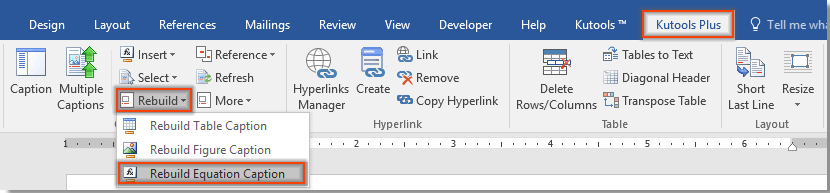#### Quickly convert text to equation captions in word

If the text is formatted as caption but it’s not a caption yet and it locates above or below the equation as shown in the below screenshot and you want to convert them to equation captions, you can quickly get it done as follows: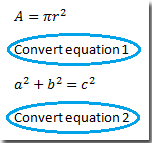1. Click Kutools Plus > Rebuild > Rebuild Equation Caption2. After applying Rebuild Equation Caption utility, there will be a dialog pop up.

If you want to convert the text above or below equation by replacing it with system equation caption style, please check Convert to system caption option, and then click OK button.

Note: Under Select Text with Number (Equation), if you click on Above option, it will choose the text above each equation and convert it to equation caption. And if you click on Below option, it will choose the text below each equation and convert it to equation caption.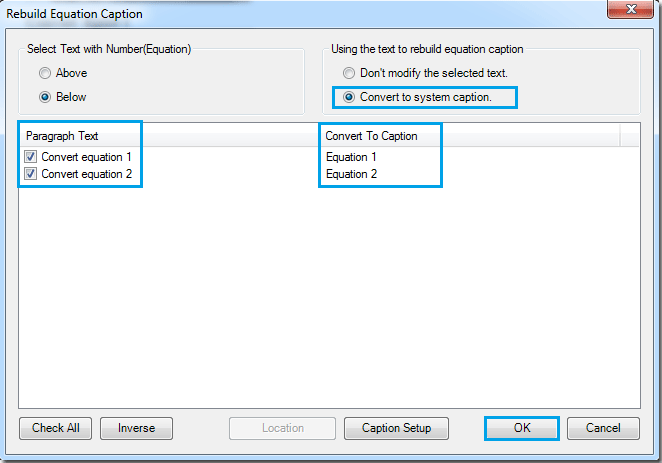If you want to convert and keep the text above or below equation as equation caption, please check Don't modify the selected text option, and then click OK button.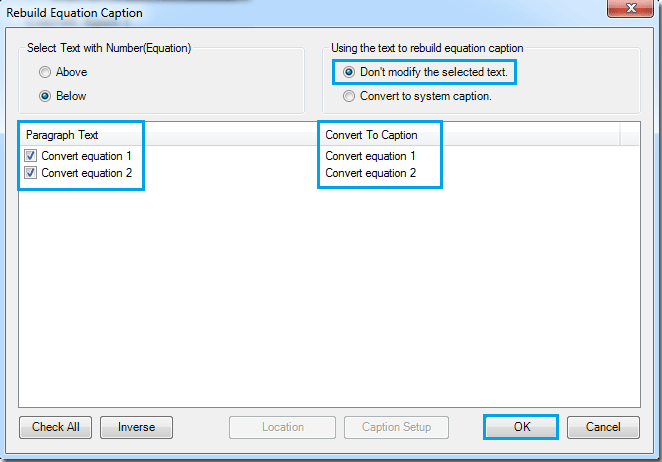3. According to the settings, you can have following two types of equation captions. See screenshots:

To convert the text above or below equation by replacing it with system equation caption style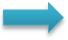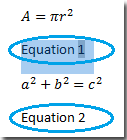To convert and keep the texts above or below equation as equation caption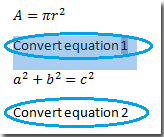Tip: If you don’t want to convert some texts above or below equations as equation caption, please uncheck them under the Paragraph Text list. See screenshot: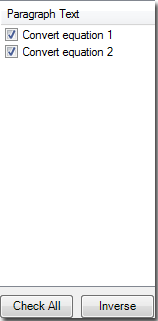### Best Office Productivity Tools

#### Kutools for Word - Elevate Your Word Experience with Over 100 Remarkable Features!

Dive into the highlighted features below or click here to explore the full power of Kutools for Word.

🔍 Precision Selections: Pinpoint specific pages  /  tables  /  shapes  /  heading paragraphs  /  Navigate with ease using our Select Group